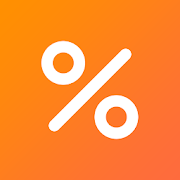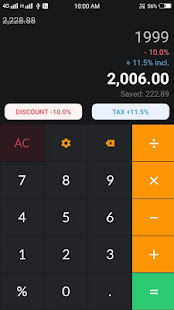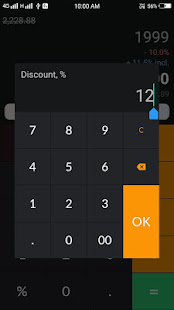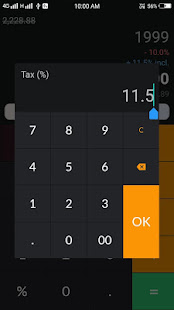# Discount and Tax Calculator

4.81 SHOPPINGDiscount calculator and tax calculator to calculate the final price after discount, amount saved, original price before discount, tax and sale price. Simple percentage calculator on discounted purchase.

Where to use discount calculator:
- Every shopping when Calculator Use. Calculate the list price, discount percentage or sale price given the other two values. You will also find the discount savings amount.
- Sale Discount Price Shopping Calculator

How to calculate discount, sale price or tax in calculator? Just follow these two simple steps and calculation will be performed automatically.
1. Set discount or tax percentage.
2. Enter amount.

In This App:
Discount calculator, Percentage calculator, Tax calculator, Simple Calculator.

Percentage discount calculator is a handy tool for determining the savings and price after discount. Use this percent discount calculator to easily calculate the final price after applying a percentage discount.

------------------------------ FAQ ------------------------------

How we calculate the Discount?
The formula for discount is exactly the same as the percentage decrease formula:
discounted_price = original_price - (original_price * discount / 100)

If you have any question about Discount calculator feel free to let us know.
Email: contactus.techplus@gmail.com.

- Minor bug fixes and performance enhancement Please write your query or suggestions to contactus.techplus@gmail.com, we would love to here from you!

### Other versions

1.0 released on 07 June 2020 (415 days ago)

### Details and Recent Ratings

Size: 6 MB
Version: 1.2 by TechPlus Developers
Updated: 10 July 2020 (382 days ago)
Released: 07 June 2020
Installations: more than 5 000
5 Stars: 94
4 Stars: 3
3 Stars: 2
2 Stars: 0
1 Star: 3

### Similar apps

Android Game Categories
Android App Categories